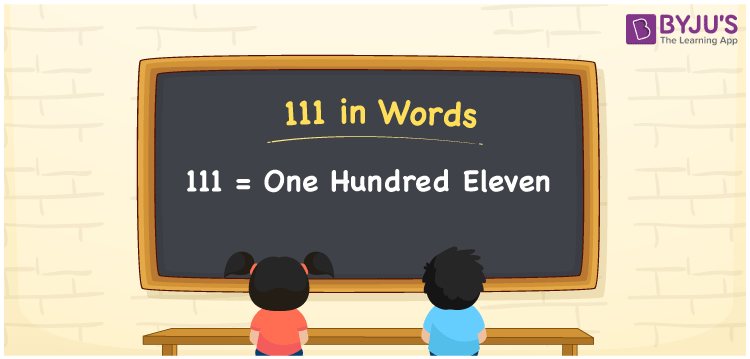# 111 in Words

We can write 111 in words as One hundred eleven. If you spotted number 111 in a newspaper, then you may read it as one hundred eleven. That means word forms of numbers are used to spell the given number or express the numerical quantity. In this article, you will understand how to convert the cardinal number 111 to words using a place value chart.

 111 in words One hundred eleven One hundred eleven in Numerical Form 111

## 111 in English Words

We generally use the English alphabet to express numbers in words. Thus, we spell 111 in English words as “One hundred eleven”.## How to Write 111 in Words?

The number 111 has three digits. Hence, we need to make a place value chart with three columns as shown below.

 Hundreds Tens Ones 1 1 1

Here, ones = 1, tens = 1, hundreds = 1

The above values can be expanded as:

1 × Hundred + 1 × Ten + 1 × One

= 100 + 10 + 1

= 100 + (10 + 1)

= 100 + 11

= One hundred + Eleven

= One hundred eleven

Therefore, 111 in words = One hundred eleven

111 is a natural number that precedes 112 and succeeds 110.

111 in words – One hundred eleven

Is 111 an even number? – No

Is 111 an odd number? – Yes

Is 111 a composite number? – No

Is 111 a prime number? – Yes

Is 111 a perfect square number? – No

Is 111 a perfect cube number? – No

## Frequently Asked Questions on 111 in Words

Q1

### How do you write 111 in words?

We can write the numeral 111 in words as One hundred eleven.
Q2

### What is the spelling of 111?

The spelling of 111 in words is One hundred eleven.
Q3

### How to write 111 in words on a cheque?

On a cheque, we write the amount 111 in words as “One hundred eleven rupees only”.
Test your Knowledge on 111 in Words